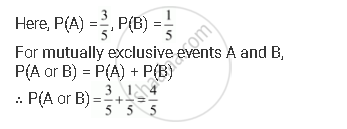CBSE (Arts) Class 11CBSE
Share

# Given P(A) = 3/5 and P(B) = 1/5 . Find P(A Or B), If a and B Are Mutually Exclusive Events. - CBSE (Arts) Class 11 - Mathematics

ConceptEvent Mutually Exclusive Events

#### Question

Given P(A) = 3/5 and P(B) = 1/5 . Find P(A or B), if A and B are mutually exclusive events.

#### SolutionIs there an error in this question or solution?

#### Video TutorialsVIEW ALL 

Solution Given P(A) = 3/5 and P(B) = 1/5 . Find P(A Or B), If a and B Are Mutually Exclusive Events. Concept: Event - Mutually Exclusive Events.
S